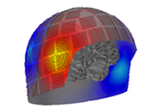# mne.viz.plot_evoked_white#

mne.viz.plot_evoked_white(evoked, noise_cov, show=True, rank=None, time_unit='s', sphere=None, axes=None, verbose=None)[source]#

Plot whitened evoked response.

Plots the whitened evoked response and the whitened GFP as described in . This function is especially useful for investigating noise covariance properties to determine if data are properly whitened (e.g., achieving expected values in line with model assumptions, see Notes below).

Parameters:
evokedinstance of `mne.Evoked`

The evoked response.

noise_cov`list` | instance of `Covariance` | path-like

The noise covariance. Can be a string to load a covariance from disk.

show`bool`

Show figure if True.

rank`None` | ‘info’ | ‘full’ | `dict`

This controls the rank computation that can be read from the measurement info or estimated from the data. When a noise covariance is used for whitening, this should reflect the rank of that covariance, otherwise amplification of noise components can occur in whitening (e.g., often during source localization).

`None`

The rank will be estimated from the data after proper scaling of different channel types.

`'info'`

The rank is inferred from `info`. If data have been processed with Maxwell filtering, the Maxwell filtering header is used. Otherwise, the channel counts themselves are used. In both cases, the number of projectors is subtracted from the (effective) number of channels in the data. For example, if Maxwell filtering reduces the rank to 68, with two projectors the returned value will be 66.

`'full'`

The rank is assumed to be full, i.e. equal to the number of good channels. If a `Covariance` is passed, this can make sense if it has been (possibly improperly) regularized without taking into account the true data rank.

`dict`

Calculate the rank only for a subset of channel types, and explicitly specify the rank for the remaining channel types. This can be extremely useful if you already know the rank of (part of) your data, for instance in case you have calculated it earlier.

This parameter must be a dictionary whose keys correspond to channel types in the data (e.g. `'meg'`, `'mag'`, `'grad'`, `'eeg'`), and whose values are integers representing the respective ranks. For example, `{'mag': 90, 'eeg': 45}` will assume a rank of `90` and `45` for magnetometer data and EEG data, respectively.

The ranks for all channel types present in the data, but not specified in the dictionary will be estimated empirically. That is, if you passed a dataset containing magnetometer, gradiometer, and EEG data together with the dictionary from the previous example, only the gradiometer rank would be determined, while the specified magnetometer and EEG ranks would be taken for granted.

The default is `None`.

time_unit`str`

The units for the time axis, can be “ms” or “s” (default).

New in v0.16.

sphere`float` | array_like | instance of `ConductorModel` | `None` | ‘auto’ | ‘eeglab’

The sphere parameters to use for the head outline. Can be array-like of shape (4,) to give the X/Y/Z origin and radius in meters, or a single float to give just the radius (origin assumed 0, 0, 0). Can also be an instance of a spherical `ConductorModel` to use the origin and radius from that object. If `'auto'` the sphere is fit to digitization points. If `'eeglab'` the head circle is defined by EEG electrodes `'Fpz'`, `'Oz'`, `'T7'`, and `'T8'` (if `'Fpz'` is not present, it will be approximated from the coordinates of `'Oz'`). `None` (the default) is equivalent to `'auto'` when enough extra digitization points are available, and (0, 0, 0, 0.095) otherwise.

New in v0.20.

Changed in version 1.1: Added `'eeglab'` option.

axes

List of axes to plot into.

New in v0.21.0.

verbose

Control verbosity of the logging output. If `None`, use the default verbosity level. See the logging documentation and `mne.verbose()` for details. Should only be passed as a keyword argument.

Returns:
figinstance of `matplotlib.figure.Figure`

The figure object containing the plot.

Notes

If baseline signals match the assumption of Gaussian white noise, values should be centered at 0, and be within 2 standard deviations (±1.96) for 95% of the time points. For the global field power (GFP), we expect it to fluctuate around a value of 1.

If one single covariance object is passed, the GFP panel (bottom) will depict different sensor types. If multiple covariance objects are passed as a list, the left column will display the whitened evoked responses for each channel based on the whitener from the noise covariance that has the highest log-likelihood. The left column will depict the whitened GFPs based on each estimator separately for each sensor type. Instead of numbers of channels the GFP display shows the estimated rank. Note. The rank estimation will be printed by the logger (if `verbose=True`) for each noise covariance estimator that is passed.

References



Engemann D. and Gramfort A. (2015) Automated model selection in covariance estimation and spatial whitening of MEG and EEG signals, vol. 108, 328-342, NeuroImage.

## Examples using `mne.viz.plot_evoked_white`#Brainstorm Elekta phantom dataset tutorial

Brainstorm Elekta phantom dataset tutorial